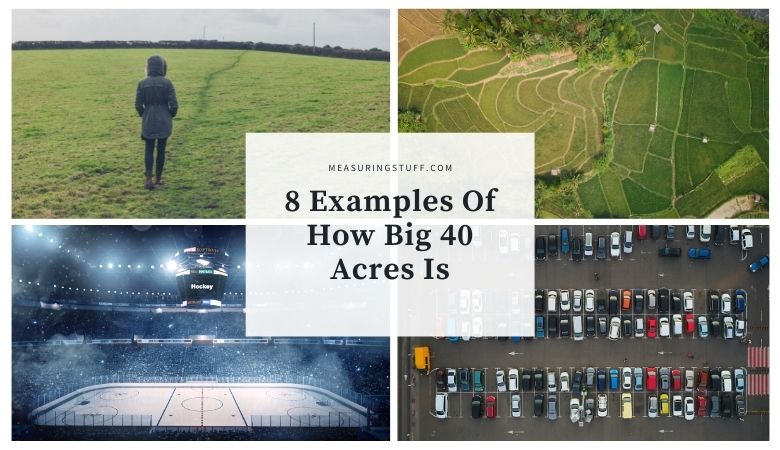# 8 Examples Of How Big 40 Acres Is (#6 Will Shock You)

Last updated on November 1st, 2022 at 10:21 pm

To get a sense of how big 40 acres really is, using some visuals can be very helpful.

An acre is a unit of measurement which is equivalent to a square measuring 66 feet by 660 feet or 43,560 square feet.

In the USA, an acre is 4,840 square meters.

40 acres would be equal to 43,560 square feet x 40 which is 1,742,400 square feet.

To visualize 40 acres, you can use the following items to actually get a sense of how big 40 acres really is.

## #1. 10,880 Parking spaces

To get a sense of how big an acre is, you can reference the size of a standard parking space.

Although the size of a parking space can vary, the standard size is 16-18 feet long and 8-9 feet wide which is about 160 square feet.

Knowing that 1 acre is equal to 43,560 square feet, simply divide this number by 160 to know how many parking spaces are in 1 acre.

In this case, there are 272 standard parking spaces in 1 acre.

To know how many parking spaces are in 40 acres, multiply 272 by 40.

242 x 40 = 10,880.

To cover an area of 40 acres, you would need to have 10,880 average-sized parking spaces.

## #2. 20 Soccer fields

If you are a soccer fan, you know how big a soccer field is. An international soccer field is between 110-120 yards long and 70-80 yards wide.

Measured in feet, a soccer field is between 330-360 feet long and 210-240 feet wide.

360 x 240 = 86,400 square feet which is equal to 1.98 acres. You can round up to 2.

2 x 20 = 40.

If you can visualize 20 soccer fields side by side, this would equal around 40 acres visually.

## #3. 40 x 1 acre of land

As mentioned, 1 acre of land is 43,560 square feet.

An example of the dimensions for 1 acre of land is 350 feet x 124.4 feet.

Many people are familiar with the size of 1 acre of land and can picture it visually

If you could see those dimensions on a map and measure it out 40 times, then you could get a sense of what 40 acres of land looks like.

## #4. 3,880 Semi – tractor trailers

If you drive frequently on main roads or highways, chances are you have seen numerous semi-trucks on the road.

The dimensions of the trailers being pulled by these trucks are either 48 or 53 feet in length and 8.5 feet wide. The 53 foot long trailers are the most common size.

To fill 1 acre of land with 53 x 8.5-foot trailers, you would need 97 of them placed side by side.

CHECK OUT  How Far Is 500 Feet?

To fill 40 acres of land with these trailers, you would need 3,880 of them.

Picturing the size of 3,880 tractor-trailers together will give you an idea of how big 40 acres really is.

## #5. 102 Ice hockey surfaces

If you are a hockey fan, you are familiar with the size of the ice surface used in the hockey arena.

Referencing a professional ice hockey surface used in leagues like the NHL, the dimensions of the ice are 200 feet long by 85 feet wide.

200 x 85 = 17,000 square feet. The size of 1 ice hockey surface is 0.39 times the size of 1 acre. In other words, less than half of 1 acre in size.

To get an idea of the size of 40 acres, you would need to visualize 102 ice hockey ice surfaces.

## #6. 15,681,600 Rulers

Most people are familiar with the size of a ruler.

But how many rulers would you need to cover 40 acres of land? 15,681,600 to be exact.

The common ruler normally used in schools and offices is exactly 12 inches long which is equal to 1 foot. They are also 1.33 inches wide.

Let’s look at this in a bit more detail.

You would need 9 rulers side by side to cover a 1-foot square that is 12 inches x 12 inches.

9 rulers = 1 square foot.

43,560 square feet = 1 acre.

43,560 x 9 = 392,040 rulers to cover 1 acre.

392,040 x 40 = 15,681,600 to cover 40 acres.

Now that’s a lot of rulers.

Basketball is one of the most popular sports played around the world. If you are familiar with the game of basketball you know how large a basketball court is.

The dimensions of a basketball court used in professional leagues like the NBA are approximately 92 feet long and 50 feet wide which equals 4,600 square feet.

To calculate this number, simply divide 43,560 (1 acre) by 4,600.

To see how many basketball courts equal 40 acres, multiply 9.5 x 40.

The answer is 380 meaning you would need this many basketball courts placed side by side to equal 40 acres.

## #8. 23 Minutes of walking time

Another great way to determine the size of 40 acres is to determine the distance you can walk over a period of time.

For example, the average person will walk approximately 3 or 4 miles per hour.

If you walk across 1 acre of land, it should take you just over 35 seconds or just over ½ minute.

If you are a slower walker, it could take up to 60 seconds or 1 minute to cross 1 acre of land.

Therefore to cover 40 acres of land while walking, it will take you around 1,400 seconds which is 23.3 minutes.

If you can walk for a time of 23.3 minutes at normal walking speed, you will cover around 40 acres of land.